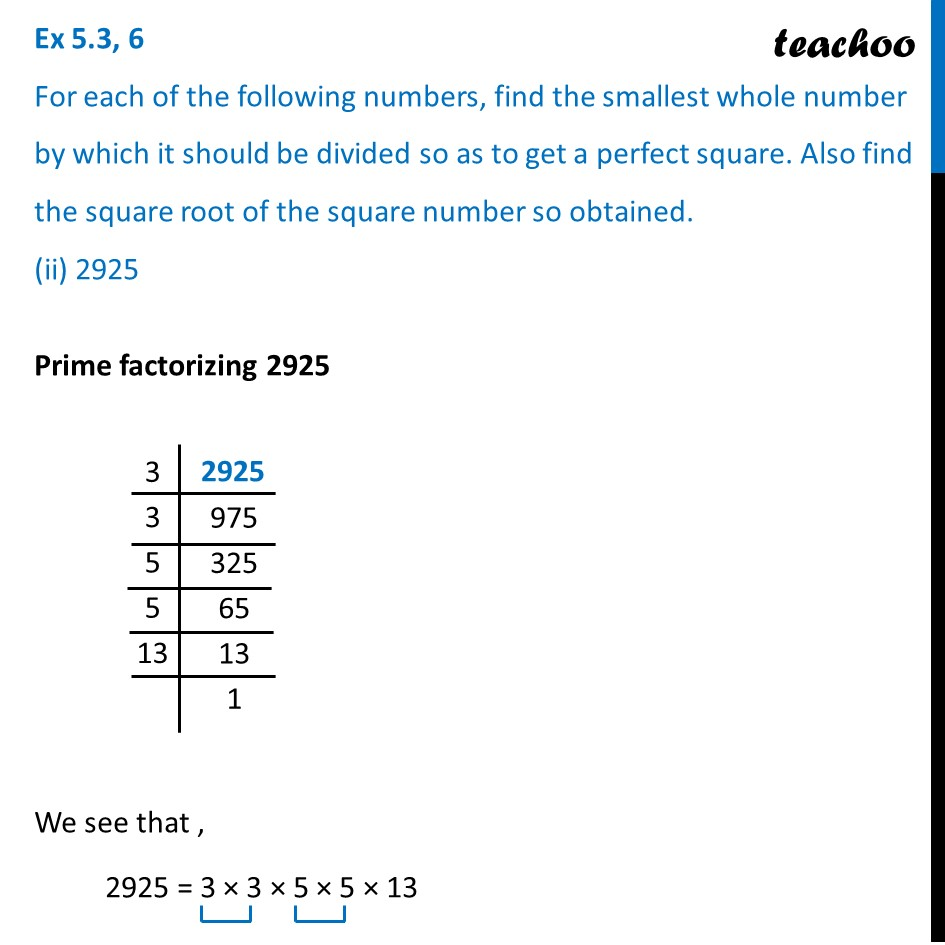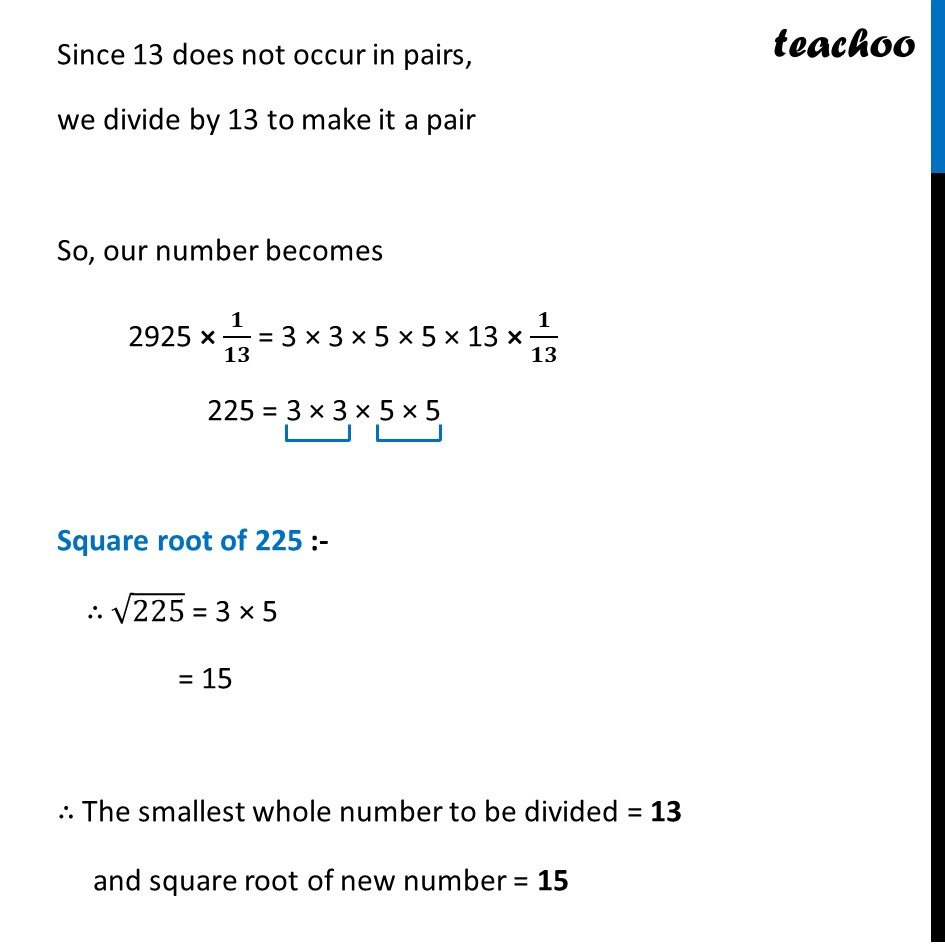Ex 5.3

Chapter 5 Class 8 Squares and Square Roots
Serial order wiseLearn in your speed, with individual attention - Teachoo Maths 1-on-1 Class

### Transcript

Ex 5.3, 6 For each of the following numbers, find the smallest whole number by which it should be divided so as to get a perfect square. Also find the square root of the square number so obtained. (iii) 396Prime factorizing 396 We see that , 396 = 2 × 2 × 3 × 3 × 11 Since 11 does not occur in pairs, we divide by 11 to make it a pair So, our number becomes 396 × 𝟏/𝟏𝟏 = 2 × 2 × 3 × 3 × 11 × 𝟏/𝟏𝟏 36 = 2 × 2 × 3 × 3 Square root of 36 :- ∴ √36 = 2 × 3 = 6 ∴ The smallest whole number to be divided = 11 and square root of new number = 6